# RD Sharma Solutions for Class 8 Math Chapter 8 - Division of Algebraic Expressions (Part-3) Notes | Study RD Sharma Solutions for Class 8 Mathematics - Class 8

## Class 8: RD Sharma Solutions for Class 8 Math Chapter 8 - Division of Algebraic Expressions (Part-3) Notes | Study RD Sharma Solutions for Class 8 Mathematics - Class 8

The document RD Sharma Solutions for Class 8 Math Chapter 8 - Division of Algebraic Expressions (Part-3) Notes | Study RD Sharma Solutions for Class 8 Mathematics - Class 8 is a part of the Class 8 Course RD Sharma Solutions for Class 8 Mathematics.
All you need of Class 8 at this link: Class 8

#### Quotient = y2−y+1 and remainder = 0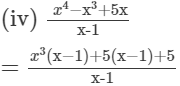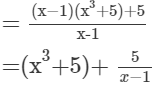Therefore, quotient =x3+5and remainder=5.(v)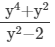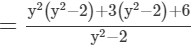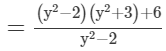=(y2+3)+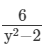Therefore,quotient=y2+3andremainder=6.Question 2: Find whether the first polynomial is a factor of the second.(i) x + 1, 2x2 + 5x + 4(ii) y − 2, 3y3 + 5y2 + 5y + 2(iii) 4x2 − 5, 4x4 + 7x2 + 15(iv) 4 − z, 3z2 − 13z + 4(v) 2a − 3, 10a2 − 9a − 5(vi) 4y + 1, 8y2 − 2y + 1Answer 2:

(i)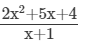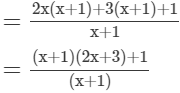=(2x+3)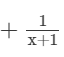∵ Remainder=1
Therefore, (x+1) is not a  factor of 2x2+5x+4
(ii)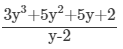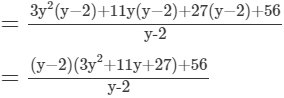=(3y2+11y+27)+ 56/y-2
∵ Remainder = 56

∴ (y−2) is not a factor of 3y3+5y2+5y+2.
(iii)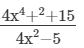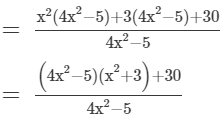=(x2+3
)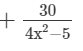∵ Remainder = 30

Therefore, (4x2−5) is not a factor of 4x4+7x2+15
(iv)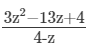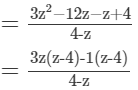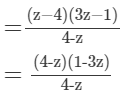=1−3z
∵ Remainder = 0
∴ (4−z) is a factor of 3z2−13z+4.
(v)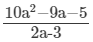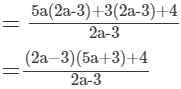=(5a+3)+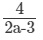∵ Remainder = 4

∴ ( 2a−3) is not a factor of 10a2−9a−5.
(vi)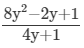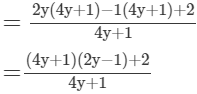=(2y−1)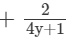∵ Remainder = 2

∴ (4y+1) is not a factor of 8y2−2y+1.
Question 3: Divide:
x2 − 5x + 6 by x − 3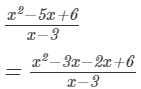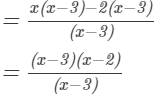= x2
Question 4:Divide:
ax2ay2 by ax + ay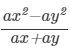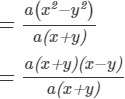= xy
Question 5: Divide:
x4y4 by x2y2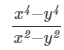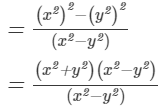= x2+y2
Question 6: Divide:
acx2 + (bc + ad)x + bd by (ax + b)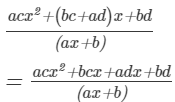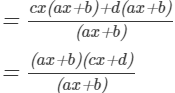= cx+d
Question 7: Divide:
(a2 + 2ab + b2) − (a2 + 2ac + c2) by 2a + b + c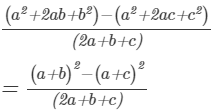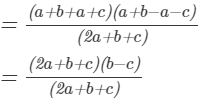=bc
Question 8: Divide: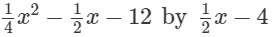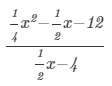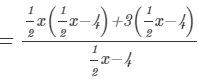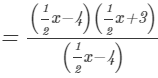=1/2 x+3

The document RD Sharma Solutions for Class 8 Math Chapter 8 - Division of Algebraic Expressions (Part-3) Notes | Study RD Sharma Solutions for Class 8 Mathematics - Class 8 is a part of the Class 8 Course RD Sharma Solutions for Class 8 Mathematics.
All you need of Class 8 at this link: Class 8Use Code STAYHOME200 and get INR 200 additional OFF

## RD Sharma Solutions for Class 8 Mathematics

88 docs

Track your progress, build streaks, highlight & save important lessons and more!

,

,

,

,

,

,

,

,

,

,

,

,

,

,

,

,

,

,

,

,

,

;